Next: Exercises Up: Incompressible Aerodynamics Previous: Ellipsoidal Airfoils

# Simple Flight Problems

Figure 9.14 shows a side-view schematic of a fixed wing aircraft flying in a straight-line at constant speed through stationary air. Here,is a horizontal coordinate, and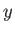a vertical coordinate. The center of mass of the aircraft is assumed to be moving with some fixed velocitythat subtends an angle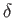with the horizontal. Thus, the wind velocity in the aircraft's rest frame is. Let the aircraft's wings, which are assumed to be parallel to its fuselage, be inclined at an angleto the horizontal. It follows that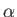is the angle of attack. The aircraft is subject to four forces: the thrust,, developed by its engine, which is assumed to act parallel to its fuselage; the lift, which acts at right-angles to; the induced drag,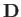, which acts in the opposite direction to; and the weight,, which acts vertically downward.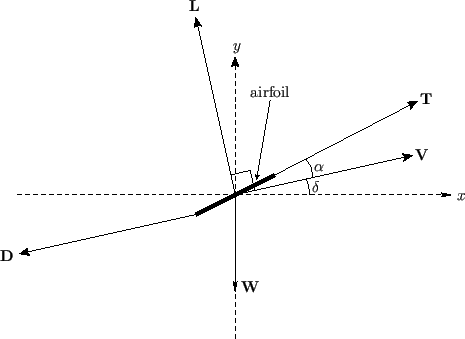Vertical force balance yields(9.111)

whereas horizontal force balance gives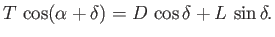(9.112)

Let us assume that the anglesandare both small. According to Equations (9.108) and (9.109),and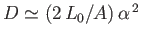. Thus,and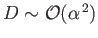. Moreover, it is clear from Equations (9.111) and (9.112) thatand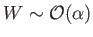. Thus, to lowest order in, Equation (9.111) yields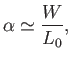(9.113)

whereas Equation (9.112) gives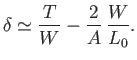(9.114)

Expression (9.113) relates the angle of attack to the ratio of the aircraft's weight to its (theoretical) maximum lift (at a given airspeed). Expression (9.114) relates the aircraft's angle of controlled (i.e., at constant airspeed) ascent to the thrust developed by its engine. An unpowered aircraft, such as a glider, has zero thrust. For such an aircraft, Equation (9.114) reveals that the angle of controlled decent--which is usually termed the glide angle--takes the value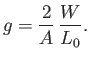(9.115)

At fixed airspeed,, and wing surface area,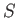, (which implies that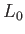is fixed) this angle can be minimized by making the wing aspect-ratio,, as large as possible. This result explains accounts for the fact that gliders (and albatrosses) have long thin wings, rather than short stubby ones. For a powered aircraft, the critical thrust to weight ratio required to maintain level flight (i.e.,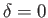) is(9.116)

Hence, this ratio is minimized by minimizing the glide angle, which explains why long-haul aircraft, which generally need to minimize fuel consumption, tend to have long thin wings. Finally, as we saw in Section 9.4, if the angle of attack exceeds some (generally small) critical valuethen boundary layer separation occurs on the back sides of the wings, giving rise to a greatly increased level of drag acting on the aircraft. In aerodynamics, this phenomenon is called a stall. As is clear from Equations (9.110) and (9.113), the requirement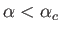is equivalent to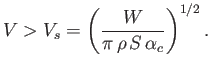(9.117)

In other words, a stall can be avoided by keeping the airspeed above the critical value, which is known as the stall speed. Note that the stall speed decreases with decreasing altitude, as the air becomes denser.Next: Exercises Up: Incompressible Aerodynamics Previous: Ellipsoidal Airfoils
Richard Fitzpatrick 2016-03-31